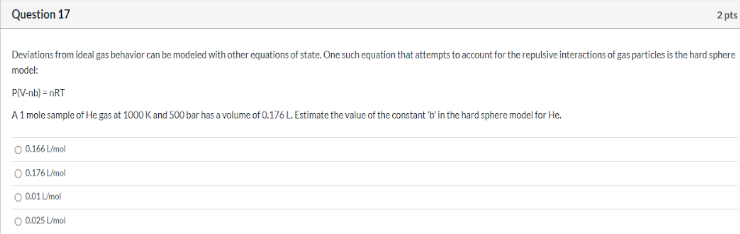# Deviations from ideal gas behavior can be modeled with other equations of state. One such equation that attempts to account for the repulsive interactions of gas particles is the hard sphere model: P(V-nb)=nRT A 1 mole sample of He gas at 1000 K and 500 bar has a volume of 0.176 L. Estimate the value of the constant "b"in the hard sphere model for He. a) 0.166 L/mol b) 0.176L/mol c) 0.01 L/mol d) 0.025 L/mol